# 1. 线性模型

$f(\boldsymbol{x}) = w_1x_1 + w_2x_2 + ... + w_dx_d +b \tag{1.1}$

$f(\boldsymbol{x}) = \boldsymbol{w}^T\boldsymbol{x}+b \tag{1.2}$

# 2. 线性回归

$f(\boldsymbol{x}_i) = \boldsymbol{w}^T\boldsymbol{x}_i+b \text{，使得} f(\boldsymbol{x}_i) \simeq y_i\tag{2.1}$

## 2.1 一元线性回归

$f(x_i) = wx_i+b\text{，使得}f(x_i)\simeq y_i \tag{2.2}$

\begin{aligned} (w^*,b^*) = \underset{(w,b)}{arg\ min}\sum_{i=1}^m(f(x_i)-y_i)^2 \\ = \underset{(w,b)}{arg\ min}\sum_{i=1}^m(y_i-wx_i-b)^2 \end{aligned} \tag{2.3}

$w^*,b^*$ 表示 $w$$b$ 的解。

$E_{(w,b)}$ 为关于 $w$$b$ 的凸函数，当它关于 $w$$b$ 的导数均为 $0$ 时，得到 $w$$b$ 的最优解。将 $E_{(w,b)}$ 分别对 $w$$b$ 求导数得：

$\frac{\partial{E_{(w,b)}}}{\partial(w)} = 2\Big(w\sum_{i=1}^m x_i^2 - \sum_{i=1}^m (y_i-b)x_i\Big) \tag{2.4}$

$\frac{\partial{E_{(w,b)}}}{\partial(b)} = 2\Big(mb - \sum_{i=1}^m (y_i-wx_i)\Big) \tag{2.5}$

$w = \frac{\sum_\limits{i=1}^m y_i(x_i-\overline{x})}{\sum\limits_{i=1}^m x_i^2 - \frac{1}{m}\Big(\sum\limits_{i=1}^m x_i\Big)^2} \tag{2.6}$

$b = \frac{1}{m}\sum_{i=1}^m (y_i-wx_i) \tag{2.7}$

$方差\ var(x) = \frac{\sum\limits_{i=1}^m(x_i-\bar{x})^2}{m-1}$

$协方差\ cov(x,y) = \frac{\sum_\limits{i=1}^m (x_i-\overline{x})(y_i-\overline{y})}{n-1}$

$w = \frac{cov(x,y)}{var(x)} = \frac{\sum_\limits{i=1}^m (x_i-\overline{x})(y_i-\overline{y})}{\sum\limits_{i=1}^m (x_i-\overline{x})^2}$

$b = \bar{y} - w\bar{x}$

# 3. 一元线性回归的Python实现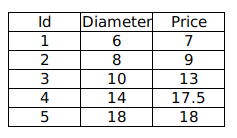## 3.1 使用 stikit-learn

### 3.1.1 导入必要模块

import matplotlib.pyplot as plt
import numpy as np
import pandas as pd
from sklearn.linear_model import LinearRegression


### 3.1.2 使用 Pandas 加载数据

pizza = pd.read_csv("pizza.csv", index_col='Id')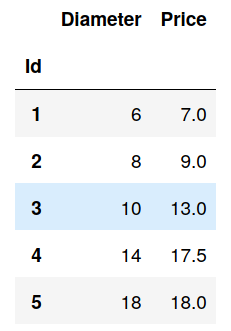### 3.1.3 快速查看数据

def runplt():
plt.figure()
plt.title("Pizza price plotted against diameter")
plt.xlabel('Diameter')
plt.ylabel('Price')
plt.grid(True)
plt.xlim(0, 25)
plt.ylim(0, 25)
return plt

dia = pizza.loc[:,'Diameter'].values
price = pizza.loc[:,'Price'].values
print(dia)
print(price)
plt = runplt()
plt.plot(dia, price, 'k.')
plt.show()

[ 6  8 10 14 18]
[ 7.   9.  13.  17.5 18. ]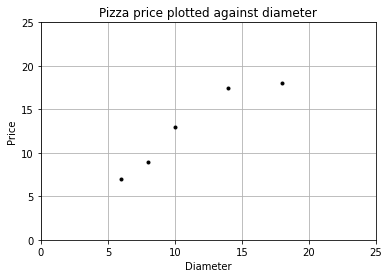### 3.1.4 使用 stlearn 创建模型

model = LinearRegression()  # 创建模型
X = dia.reshape((-1,1))
y = price
model.fit(X, y)  # 拟合

X2 = [, ] # 取两个预测值
y2 = model.predict(X2)  # 进行预测
print(y2)  # 查看预测值

plt = runplt()
plt.plot(dia, price, 'k.')
plt.plot(X2, y2, 'g-')  # 画出拟合曲线
plt.show()

[ 1.96551724 26.37284483]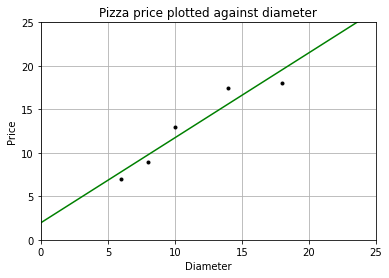fit() 的第一个参数 X 为 shape(样本个数，属性个数) 的数组或矩阵类型的参数，代表输入空间；

### 3.1.5 模型评估

plt = runplt()
plt.plot(dia, price, 'k.')
plt.plot(X2, y2, 'g-')

# 画出残差
yr = model.predict(X)
for index, x in enumerate(X):
plt.plot([x, x], [y[index], yr[index]], 'r-')

plt.show()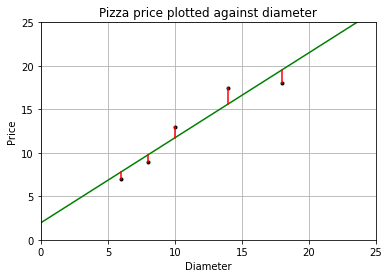print("均方误差为: %.2f" % np.mean((model.predict(X)-y) ** 2))

均方误差为: 1.75


## 3.2 手动实现

### 3.2.1 计算 w 和 b

$w$$b$ 的最优解的闭式（closed-form）解为：

$w = \frac{\sum_\limits{i=1}^m y_i(x_i-\overline{x})}{\sum\limits_{i=1}^m x_i^2 - \frac{1}{m}\Big(\sum\limits_{i=1}^m x_i\Big)^2} \tag{2.6}$

$b = \frac{1}{m}\sum_{i=1}^m (y_i-wx_i) \tag{2.7}$

$方差\ var(x) = \frac{\sum\limits_{i=1}^m(x_i-\bar{x})^2}{m-1}$

$协方差\ cov(x,y) = \frac{\sum_\limits{i=1}^m (x_i-\overline{x})(y_i-\overline{y})}{n-1}$

$w = \frac{cov(x,y)}{var(x)} = \frac{\sum_\limits{i=1}^m (x_i-\overline{x})(y_i-\overline{y})}{\sum\limits_{i=1}^m (x_i-\overline{x})^2}$

$b = \bar{y} - w\bar{x}$

# 法一：
# w = np.sum(price * (dia - np.mean(dia))) / (np.sum(dia**2) - (1/dia.size) * (np.sum(dia))**2)
# b = (1 / dia.size) * np.sum(price - w * dia)

#法二：
variance = np.var(dia, ddof=1)  # 计算方差，doff为贝塞尔（无偏估计）校正系数
covariance = np.cov(dia, price)  # 计算协方差
w = covariance / variance
b = np.mean(price) - w * np.mean(dia)

print("w = %f\nb = %f" % (w, b))

y_pred = w * dia + b

plt = runplt()
plt.plot(dia, price, 'k.')  # 样本点
plt.plot(dia, y_pred, 'b-')  # 手动求出的线性回归模型
plt.plot(X2, y2, 'g-.')  # 使用LinearRegression.fit()求出的模型
plt.show()

w = 0.976293
b = 1.965517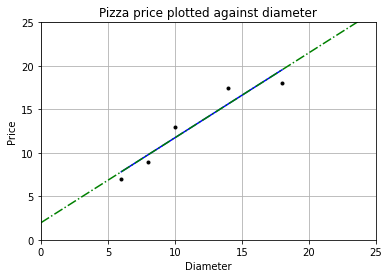### 3.2.2 功能封装

class LinearRegression:
"""
拟合一元线性回归模型

Parameters
----------
x : shape 为(样本个数,)的 numpy.array
只有一个属性的数据集

y : shape 为(样本个数,)的 numpy.array
标记空间

Returns
-------
self : 返回 self 的实例.
"""
def __init__(self):
self.w = None
self.b = None

def fit(self, x, y):
variance = np.var(x, ddof=1)  # 计算方差，doff为贝塞尔（无偏估计）校正系数
covariance = np.cov(x, y)  # 计算协方差
self.w = covariance / variance
self.b = np.mean(y) - w * np.mean(x)
#         self.w = np.sum(y * (x - np.mean(x))) / (np.sum(x**2) - (1/x.size) * (np.sum(x))**2)
#         self.b = (1 / x.size) * np.sum(y - self.w * x)
return self

def predict(self, x):
"""
使用该线性模型进行预测

Parameters
----------
x : 数值 或 shape 为(样本个数,)的 numpy.array
属性值

Returns
-------
C : 返回预测值
"""
return self.w * x + self.b


# 创建并拟合模型
model = LinearRegression()
model.fit(dia, price)

x2 = np.array([0, 25])  # 取两个预测值
y2 = model.predict(x2)  # 进行预测
print(y2)  # 查看预测值

runplt()
plt.plot(dia, price, 'b.')
plt.plot(x2, y2, 'y-')  # 画出拟合
plt.show()

[ 1.96551724 26.37284483]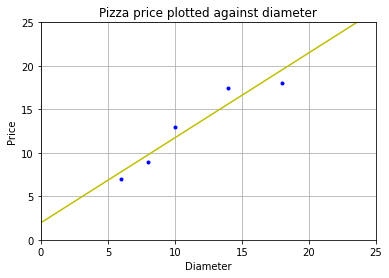posted @ 2020-03-31 15:56  Raina_RLN  阅读(1900)  评论(0编辑  收藏